ArcGIS Developer
Dashboard

# Correlation - Hurricane analysis, part 3/3¶

This is the third part to a three part set of notebooks that process and analyze historic hurricane tracks. In the previous notebooks we saw:

Part 1

2. Cleaning and merging hurricane observations using DASK
3. Aggregating point observations into hurricane tracks using ArcGIS GeoAnalytics server

Part 2

1. Visualize the locations of hurricane tracks
2. Different basins and the number of hurricanes per basin
3. Number of hurricanes over time
4. Seasonality in occurrence of hurricanes
5. Places where hurricanes make landfall and the people are affected

In this notebook you will analyze the aggregated tracks to answer important questions about hurricane severity and how they correlate over time.

Import the libraries necessary for this notebook.

In :
```# import ArcGIS Libraries
from arcgis.gis import GIS
from arcgis.geometry import filters
from arcgis.geocoding import geocode
from arcgis.features.manage_data import overlay_layers
from arcgis.geoenrichment import enrich

# import Pandas for data exploration
import pandas as pd
import numpy as np
from scipy import stats

# import plotting libraries
import matplotlib.pyplot as plt
import seaborn as sns
%matplotlib inline

# import display tools
from pprint import pprint
from IPython.display import display

# import system libs
from sys import getsizeof

# Miscellaneous imports
import warnings
warnings.filterwarnings('ignore')
```
In :
```gis = GIS('https://pythonapi.playground.esri.com/portal', 'arcgis_python', 'amazing_arcgis_123')
```

## Access aggregated hurricane data¶

Below, we access the tracks aggregated using GeoAnalytics in the previous notebook.

In :
```hurricane_tracks_item = gis.content.search('title:hurricane_tracks_aggregated_ga', item_type="feature layer")
hurricane_fl = hurricane_tracks_item.layers
```

The GeoAnalytics step calculated summary statistics of all numeric fields. However, only a few of the columns are of interest to us.

In :
```pprint([f['name'] for f in hurricane_fl.properties.fields], compact=True, width=80)
```
```['objectid', 'serial_num', 'count', 'count_col_1', 'sum_col_1', 'min_col_1',
'max_col_1', 'mean_col_1', 'range_col_1', 'sd_col_1', 'var_col_1',
'count_season', 'sum_season', 'min_season', 'max_season', 'mean_season',
'range_season', 'sd_season', 'var_season', 'count_num', 'sum_num', 'min_num',
'max_num', 'mean_num', 'range_num', 'sd_num', 'var_num', 'count_basin',
'any_basin', 'count_sub_basin', 'any_sub_basin', 'count_name', 'any_name',
'count_iso_time', 'any_iso_time', 'count_nature', 'any_nature', 'count_center',
'any_center', 'count_track_type', 'any_track_type', 'count_current_basin',
'any_current_basin', 'count_latitude_merged', 'sum_latitude_merged',
'min_latitude_merged', 'max_latitude_merged', 'mean_latitude_merged',
'range_latitude_merged', 'sd_latitude_merged', 'var_latitude_merged',
'count_longitude_merged', 'sum_longitude_merged', 'min_longitude_merged',
'max_longitude_merged', 'mean_longitude_merged', 'range_longitude_merged',
'sd_longitude_merged', 'var_longitude_merged', 'count_wind_merged',
'sum_wind_merged', 'min_wind_merged', 'max_wind_merged', 'mean_wind_merged',
'range_wind_merged', 'sd_wind_merged', 'var_wind_merged',
'count_pressure_merged', 'sum_pressure_merged', 'min_pressure_merged',
'max_pressure_merged', 'mean_pressure_merged', 'range_pressure_merged',
'min_eye_dia_merged', 'max_eye_dia_merged', 'mean_eye_dia_merged',
'range_eye_dia_merged', 'sd_eye_dia_merged', 'var_eye_dia_merged',
'track_duration', 'end_datetime', 'start_datetime']
```

Below we select the following fields for the rest of this analysis.

In :
```fields_to_query = ['objectid', 'count', 'min_season', 'any_basin', 'any_sub_basin',
'any_name', 'mean_latitude_merged', 'mean_longitude_merged',
'max_wind_merged', 'range_wind_merged', 'min_pressure_merged',
'range_pressure_merged', 'max_eye_dia_merged', 'track_duration',
'end_datetime', 'start_datetime']
```

### Query hurricane tracks into a Spatially Enabled `DataFrame`¶

In :
```all_hurricanes_df = hurricane_fl.query(out_fields=','.join(fields_to_query), as_df=True)
```
```CPU times: user 1.12 s, sys: 318 ms, total: 1.43 s
Wall time: 4.5 s
```
In :
```all_hurricanes_df.shape
```
Out:
`(12362, 17)`

There are `12,362` hurricanes identified by GeoAnalytics aggregate tracks tool. To get an idea about this aggregated dataset, call the `head()` method.

In :
```all_hurricanes_df.head()
```
Out:
SHAPE any_basin any_name any_sub_basin count end_datetime max_eye_dia_merged max_wind_merged mean_latitude_merged mean_longitude_merged min_pressure_merged min_season objectid range_pressure_merged range_wind_merged start_datetime track_duration
0 {"paths": [[[59.60000000000002, -17.6000000000... SI NOT NAMED MM 7.0 1854-02-10 18:00:00 NaN NaN -19.318571 60.639286 NaN 1854.0 1 NaN NaN 1854-02-08 06:00:00 1.296000e+08
1 {"paths": [[[-23.5, 12.5], [-24.19999999999999... NA NOT NAMED NA 9.0 1859-08-26 12:00:00 NaN 45.0 14.000000 -26.222222 NaN 1859.0 2 NaN 10.0 1859-08-24 12:00:00 1.728000e+08
2 {"paths": [[[-23.19999999999999, 12.1000000000... NA UNNAMED NA 50.0 1853-09-12 18:00:00 NaN 130.0 26.982000 -51.776000 924.0 1853.0 3 53.0 90.0 1853-08-30 00:00:00 1.058400e+09
3 {"paths": [[[59.80000000000001, -15.5], [59.49... SI XXXX856017 MM 13.0 1856-04-05 18:00:00 NaN NaN -20.185385 59.573077 NaN 1856.0 4 NaN NaN 1856-04-02 18:00:00 2.592000e+08
4 {"paths": [[[99.60000000000002, -11.5], [98.30... SI NOT NAMED WA 13.0 1861-03-15 18:00:00 NaN NaN -12.940769 94.183846 NaN 1861.0 5 NaN NaN 1861-03-12 18:00:00 2.592000e+08

To better analyze this data set, the date columns need to be changed to a format that Pandas understands better. This is accomplished by calling `to_datetime()` method and passing the appropriate time columns.

In :
```all_hurricanes_df['start_datetime'] = pd.to_datetime(all_hurricanes_df['start_datetime'])
all_hurricanes_df['end_datetime'] = pd.to_datetime(all_hurricanes_df['end_datetime'])
all_hurricanes_df.index = all_hurricanes_df['start_datetime']
```
Out:
SHAPE any_basin any_name any_sub_basin count end_datetime max_eye_dia_merged max_wind_merged mean_latitude_merged mean_longitude_merged min_pressure_merged min_season objectid range_pressure_merged range_wind_merged start_datetime track_duration
start_datetime
1854-02-08 06:00:00 {"paths": [[[59.60000000000002, -17.6000000000... SI NOT NAMED MM 7.0 1854-02-10 18:00:00 NaN NaN -19.318571 60.639286 NaN 1854.0 1 NaN NaN 1854-02-08 06:00:00 1.296000e+08
1859-08-24 12:00:00 {"paths": [[[-23.5, 12.5], [-24.19999999999999... NA NOT NAMED NA 9.0 1859-08-26 12:00:00 NaN 45.0 14.000000 -26.222222 NaN 1859.0 2 NaN 10.0 1859-08-24 12:00:00 1.728000e+08
1853-08-30 00:00:00 {"paths": [[[-23.19999999999999, 12.1000000000... NA UNNAMED NA 50.0 1853-09-12 18:00:00 NaN 130.0 26.982000 -51.776000 924.0 1853.0 3 53.0 90.0 1853-08-30 00:00:00 1.058400e+09
1856-04-02 18:00:00 {"paths": [[[59.80000000000001, -15.5], [59.49... SI XXXX856017 MM 13.0 1856-04-05 18:00:00 NaN NaN -20.185385 59.573077 NaN 1856.0 4 NaN NaN 1856-04-02 18:00:00 2.592000e+08
1861-03-12 18:00:00 {"paths": [[[99.60000000000002, -11.5], [98.30... SI NOT NAMED WA 13.0 1861-03-15 18:00:00 NaN NaN -12.940769 94.183846 NaN 1861.0 5 NaN NaN 1861-03-12 18:00:00 2.592000e+08

The track duration and length columns need to be projected to units (days, hours, miles) that are meaningful for analysis.

In :
```all_hurricanes_df['track_duration_hrs'] = all_hurricanes_df['track_duration'] / 3600000
all_hurricanes_df['track_duration_days'] = all_hurricanes_df['track_duration'] / (3600000*24)
```

### Query landfall tracks layer into a Spatially Enabled `DataFrame`¶

We query the landfall tracks layer created in the pervious notebook into a DataFrame.

In :
```inland_tracks = gis.content.search('hurricane_landfall_tracks')

fields_to_query = ['min_season', 'any_basin','any_name', 'max_wind_merged',
'min_pressure_merged', 'track_duration','end_datetime',
'start_datetime', 'analysislength']

landfall_tracks_fl = inland_tracks.layers
```
In :
```landfall_tracks_df = landfall_tracks_fl.query(out_fields=fields_to_query).df
```
Out:
analysislength any_basin any_name end_datetime max_wind_merged min_pressure_merged min_season objectid start_datetime track_duration SHAPE
0 4.376642 NA NOT NAMED -3663424800000 95.0 965.0 1853.0 1 -3664699200000 1.317600e+09 {'paths': [[[-74.47272727299998, 24], [-74.463...
1 117.097286 NA UNNAMED -3645172800000 70.0 NaN 1854.0 2 -3645475200000 2.160000e+08 {'paths': [[[-99.13749999999999, 26.5699999999...
2 256.909588 NA UNNAMED -3645172800000 70.0 NaN 1854.0 3 -3645475200000 2.160000e+08 {'paths': [[[-102.21739130399999, 27.686956522...

## Manage missing sensor data¶

Before we can analyze if hurricanes intensify over time, we need to identify and account for missing values in our data. Sensor measurements such as wind speed, atmospheric pressure, eye diameter, generally suffer from missing values and outliers. The reconstruct tracks tool has identified `12,362` individual hurricanes that occurred during the past `169` years.

In :
```all_hurricanes_df.shape
```
Out:
`(12362, 19)`

### Visualize missing records¶

An easy way to visualize missing records is to hack the `heatmap` of `seaborn` library to display missing records. The snippet below shows missing records in yellow color.

In :
```missing_data_viz = all_hurricanes_df.replace(0,np.NaN)
missing_data_viz = missing_data_viz.replace(-9999.0,np.NaN)
missing_data_viz['min_pressure_merged'] = missing_data_viz['min_pressure_merged'].replace(100.0,np.NaN)

plt.figure(figsize=(10,10))
missing_data_ax = sns.heatmap(missing_data_viz[['max_wind_merged', 'min_pressure_merged',
'max_eye_dia_merged', 'track_duration']].isnull(),
cbar=False, cmap='viridis')
missing_data_ax.set_ylabel('Years')
missing_data_ax.set_title('Missing values (yellow) visualized as a heatmap')
```
Out:
`Text(0.5,1,'Missing values (yellow) visualized as a heatmap')`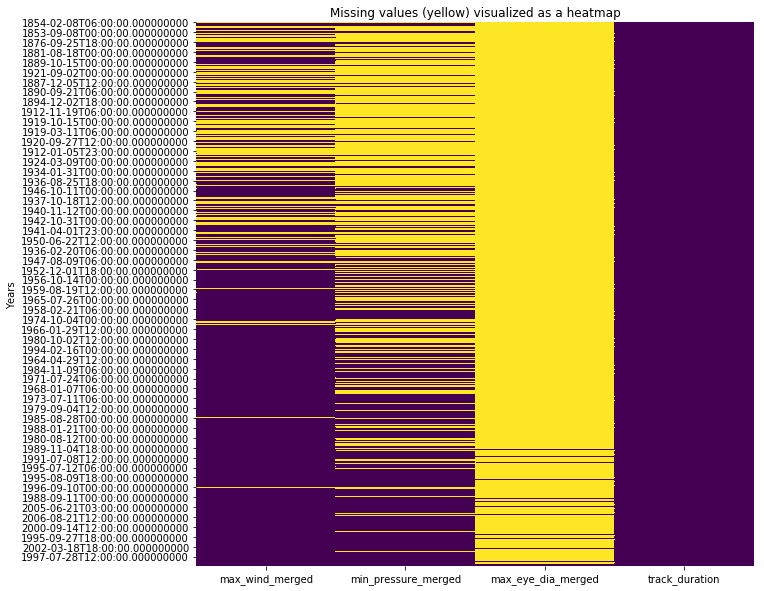All three observation columns - wind speed, atmospheric pressure and eye diameter, suffer from missing values. In general as technology improved over time, we were able to collect better data with fewer missing observations. In the sections below, we attempt to fill these values using different techniques. We will compare how they fare and pick one of them for rest of the analysis.

### Missing value imputation¶

Technique 1: Drop missing values: An easy way to deal with missing values is to drop those record from analysis. If we were to do that, we lose over a third of the hurricanes.

In :
```hurricanes_nona = missing_data_viz.dropna(subset=['max_wind_merged','min_pressure_merged'])
hurricanes_nona.shape
```
Out:
`(5857, 19)`

Technique 2: Fill using median value: A common technique is to fill using median value (or a different measure of centrality). This technique computes the median of the entire column and applies that to all the missing values.

In :
```fill_values = {'max_wind_merged': missing_data_viz['max_wind_merged'].median(),
'min_pressure_merged': missing_data_viz['min_pressure_merged'].median(),
'track_duration_hrs': missing_data_viz['track_duration_hrs'].median()}
hurricanes_fillna = missing_data_viz.fillna(value=fill_values)
```

Technique 3: Fill by interpolating between existing values: A sophisticated approach is to interploate a missing value based on two of its closest observations.

In :
```hurricanes_ipl = missing_data_viz
hurricanes_ipl['max_wind_merged'] = hurricanes_ipl['max_wind_merged'].interpolate()
hurricanes_ipl['min_pressure_merged'] = hurricanes_ipl['min_pressure_merged'].interpolate()
hurricanes_ipl['track_duration_hrs'] = hurricanes_ipl['track_duration_hrs'].interpolate()
```

Visualize all 3 techniques

To compare how each of these techniques fared, we will plot the histogram of wind speed column after managing for missing values.

In :
```fig, ax = plt.subplots(1,3, sharex=True, figsize=(15,5))
fig.suptitle('Comparing effects of missing value imputations on Wind speed column',
fontsize=15)

hurricanes_nona['max_wind_merged'].plot(kind='hist', ax=ax, bins=35, title='Drop null values')
hurricanes_fillna['max_wind_merged'].plot(kind='hist', ax=ax, bins=35, title='Impute with median')
hurricanes_ipl['max_wind_merged'].plot(kind='hist', ax=ax, bins=35, title='Impute via interpolation')
for a in ax:
a.set_xlabel('Wind Speed')
```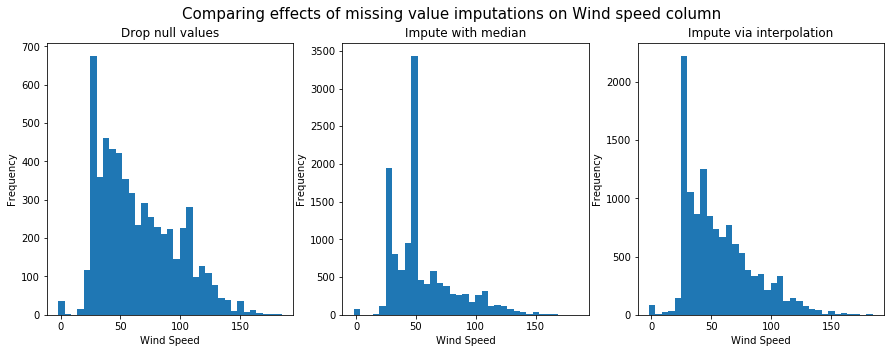Next, we will plot the histogram of atmospheric pressure column after managing for missing values.

In :
```fig, ax = plt.subplots(1,3, sharex=True, figsize=(15,5))
fig.suptitle('Comparing effects of missing value imputations on Pressure column',
fontsize=15)

hurricanes_nona['min_pressure_merged'].plot(kind='hist', ax=ax, title='Drop null values')
hurricanes_fillna['min_pressure_merged'].plot(kind='hist', ax=ax, title='Impute with median')
hurricanes_ipl['min_pressure_merged'].plot(kind='hist', ax=ax, title='Impute via interpolation')
for a in ax:
a.set_xlabel('Atmospheric Pressure')
```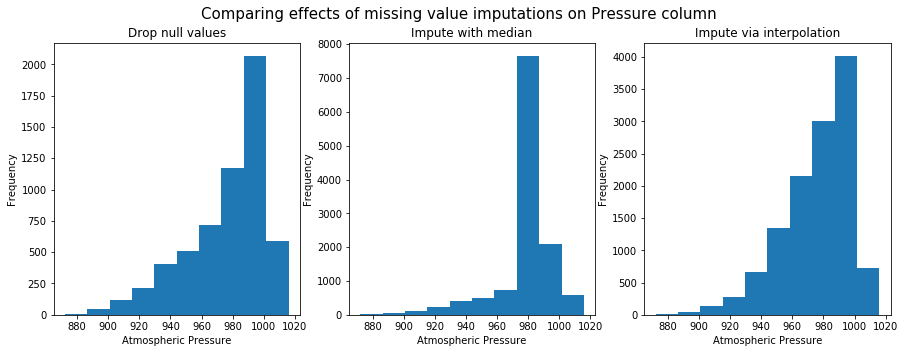Fill using interpolation preserves shape of the original distribution. So it will be used for further anlaysis.

# Does intensity of hurricanes increase over time?¶

This last part of this study analyzes if there a temporal trend in the intensity of hurricanes. A number of studies have concluded that anthropogenic influences in the form of global climate change make hurricanes worse and dangerous. We analyze if such patterns can be noticed from an empirical standpoint.

## Does the number of hurricanes increase over time?¶

In :
```ax = all_hurricanes_df['min_season'].hist(bins=50)
ax.set_title('Number of hurricanes per season')
```
Out:
`Text(0.5,1,'Number of hurricanes per season')`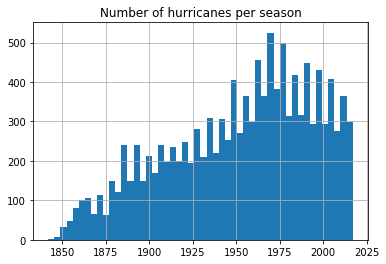From the previous notebook, we noticed the number of hurricanes recorded has been steadily increasing, partly due to advancements in technology. We notice a reduction in number of hurricanes after 1970s. Let us split this up by basin and observe if the trend is similar.

In :
```fgrid = sns.FacetGrid(data=all_hurricanes_df, col='any_basin', col_wrap=3,
sharex=False, sharey=False)
fgrid.map(plt.hist, 'min_season', bins=50)
fgrid.set_axis_labels(x_var='Seasons', y_var='Frequency of hurricanes')
```
Out:
`<seaborn.axisgrid.FacetGrid at 0x1a35c9f908>`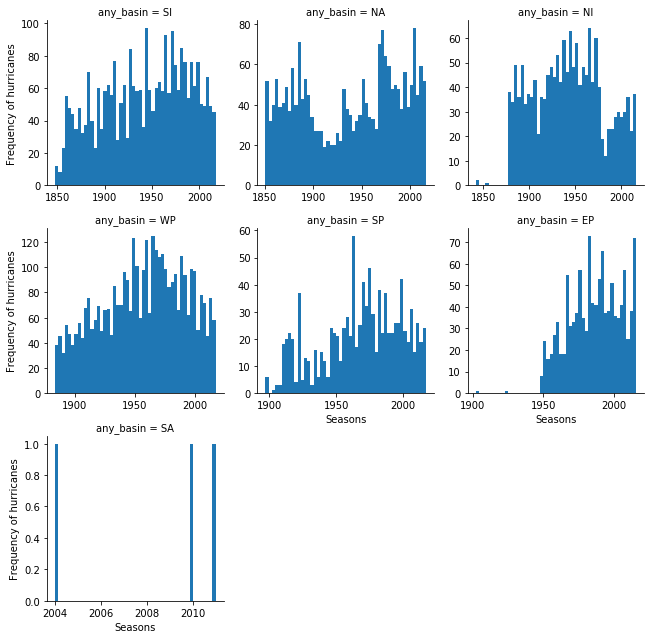Plotting the frequency of hurricanes by basin shows a similar trend with the number of hurricanes reducing globally after 1970s. This is consistent with certain studies (1). However, this is only one part of the story. Below, we continue to analyze if the nature of hurricanes itself is changing, while the total number may reduce.

## Does hurricane wind speed increase over time?¶

To understand if wind speed increases over time, we create a scatter plot of `min_season` against the `max_wind_merged` column. The `seaborn` plotting library can enhance this plot with a correlation coefficient and its level of signifance (p value).

In :
```jgrid = sns.jointplot(x='min_season', y='max_wind_merged', data=hurricanes_ipl,
kind='reg', joint_kws={'line_kws':{'color':'green'}}, height=7, space=0.5)
j = jgrid.annotate(stats.pearsonr)
j = jgrid.ax_joint.set_title('Does hurricane wind speed increase over time?')
```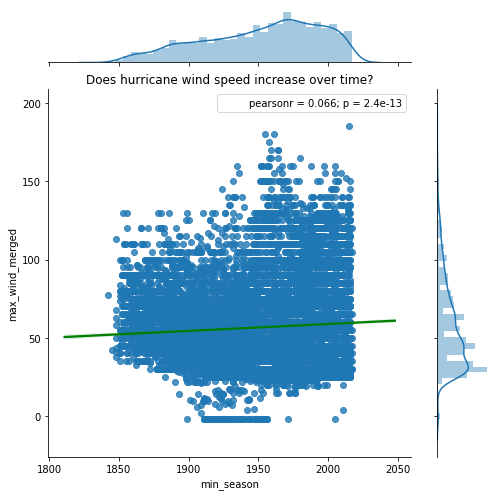From the plot above, we notice a small positive correlation. Wind speeds are observed to increase with time. The small `p-value` suggests this correlation (albeit small) is statistically significant. The plot above considers hurricanes across all the basins and regresses that against time. To get a finer picture, we need to split the data by basins and observe the correlation.

In :
```# since there are not many hurricanes observed over South Atlantic basin (SA),
# we drop it from analysis
hurricanes_major_basins_df = hurricanes_ipl[hurricanes_ipl['any_basin'].isin(
['WP','SI','NA','EP','NI','SP'])]
```

Define a function that can compute `pearson-r` correlation coefficient for any two columns across all basins.

In :
```def correlate_by_basin(column_a, column_b, df=hurricanes_major_basins_df,
category_column = 'any_basin'):
# clean data by dropping any NaN values
df_nona = df.dropna(subset=[column_a, column_b])

# loop through the basins
basins = list(df[category_column].unique())
return_dict = {}
for basin in basins:
subset_df = df_nona[df_nona[category_column] == basin]
r, p = stats.pearsonr(list(subset_df[column_a]), list(subset_df[column_b]))

return_dict[basin] = [round(r,4), round(p,4)]

# return correlation coefficient and p-value for each basin as a DataFrame
return_df = pd.DataFrame(return_dict).T
return_df.columns=['pearson-r','p-value']
return return_df
```

### Analyzing hurricane wind speed over time by basin¶

Below we plot a grid of scatter plots with linear regression plots overlaid over them. The `seaborn` library's `lmplot()` function makes it trivial to accomplish this in a single command.

In :
```fgrid = sns.lmplot('min_season', 'max_wind_merged', col='any_basin',
data=hurricanes_major_basins_df, col_wrap=3,
sharex=False, sharey=False, line_kws={'color':'green'})
```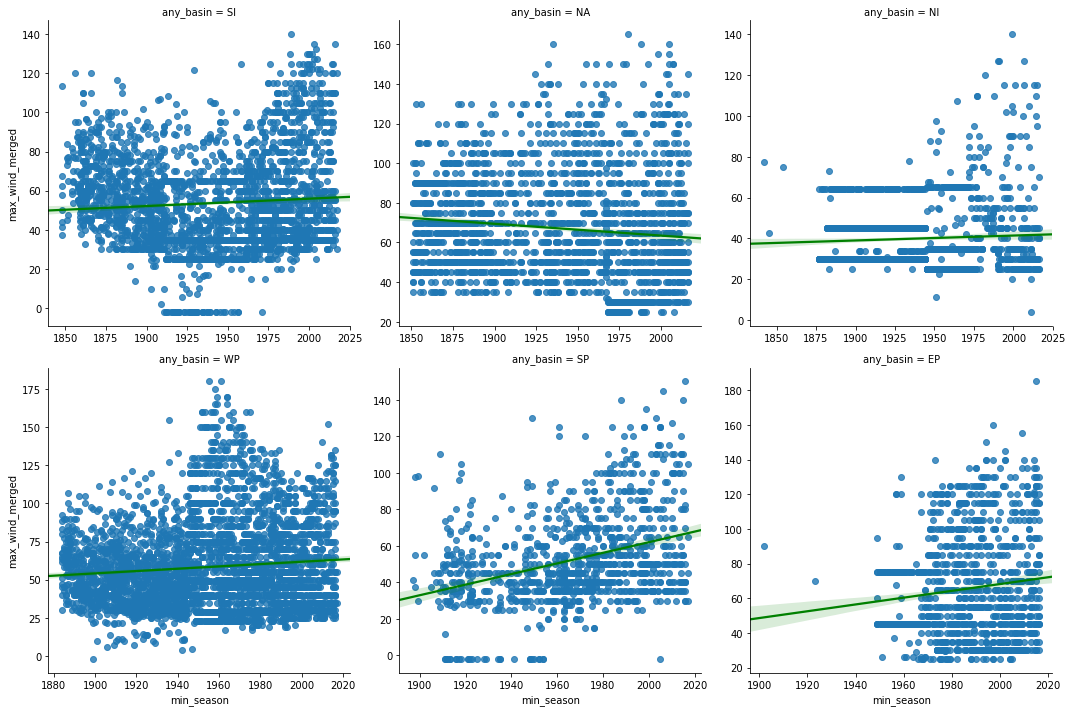From the scatter plots above, we notice the wind speeds in most basins show a slight positive trend, with North Atlantic being an exception. To explore this further, we compute the correlation coefficient and its p-value below.

In :
```wind_vs_season = correlate_by_basin('min_season','max_wind_merged')
print('Correlation coefficients for min_season vs max_wind_merged')
wind_vs_season
```
```Correlation coefficients for min_season vs max_wind_merged
```
Out:
pearson-r p-value
SI 0.0686 0.0003
NA -0.1073 0.0000
NI 0.0528 0.0357
WP 0.0891 0.0000
SP 0.3415 0.0000
EP 0.1177 0.0001

The table above displays the correlation coefficient of hurricane wind speed over time. Hurricanes over the Southern Pacific basin exhibit a positive trend of increasing wind speeds. The `r` value over the North Atlantic shows a weak negative trend. Since all the `p-value`s are less than `0.05`, these correlations are statistically significant.

### Analyzing hurricane category over time by basin¶

Hurricanes are classified on a Saffir-Simpson scale of `1-5` based on their wind speed. Let us compute this column on the dataset and observe if there are temporal aspects to it.

In :
```def categorize_hurricanes(row, wind_speed_column='max_wind_merged'):
wind_speed = row[wind_speed_column] * 1.152  # knots to mph
if 74 <= wind_speed <= 95:
return '1'
elif 96 <= wind_speed <= 110:
return '2'
elif 111 <= wind_speed <= 129:
return '3'
elif 130 <= wind_speed <= 156:
return '4'
elif 157 <= wind_speed <= 500:
return '5'
```
In :
```hurricanes_major_basins_df['category_str'] = hurricanes_major_basins_df.apply(categorize_hurricanes,
axis=1)
hurricanes_major_basins_df['category'] = pd.to_numeric(arg=hurricanes_major_basins_df['category_str'],
errors='coerce', downcast='integer')

```
Out:
SHAPE any_basin any_name any_sub_basin count end_datetime max_eye_dia_merged max_wind_merged mean_latitude_merged mean_longitude_merged ... min_season objectid range_pressure_merged range_wind_merged start_datetime track_duration track_duration_hrs track_duration_days category category_str
start_datetime
1854-02-08 06:00:00 {'paths': [[[59.60000000000002, -17.6000000000... SI NOT NAMED MM 7.0 1854-02-10 18:00:00 NaN NaN -19.318571 60.639286 ... 1854.0 1 NaN NaN 1854-02-08 06:00:00 129600000.0 36.0 1.5 NaN None
1859-08-24 12:00:00 {'paths': [[[-23.5, 12.5], [-24.19999999999999... NA NOT NAMED NA 9.0 1859-08-26 12:00:00 NaN 45.0 14.000000 -26.222222 ... 1859.0 2 NaN 10.0 1859-08-24 12:00:00 172800000.0 48.0 2.0 NaN None

2 rows × 21 columns

We will create violin and bar plots to visualize the number of hurricane categories over different basins.

In :
```fig, ax = plt.subplots(1,2, figsize=(15,6))
vplot = sns.violinplot(x='any_basin', y='category', data=hurricanes_major_basins_df, ax=ax)
vplot.set_title('Number of hurricanes per category in each basin')

cplot = sns.countplot(x='any_basin', hue='category_str', data=hurricanes_major_basins_df,
hue_order=['1','2','3','4','5'], ax=ax)
cplot.set_title('Number of hurricanes per category in each basin')
```
Out:
`Text(0.5,1,'Number of hurricanes per category in each basin')`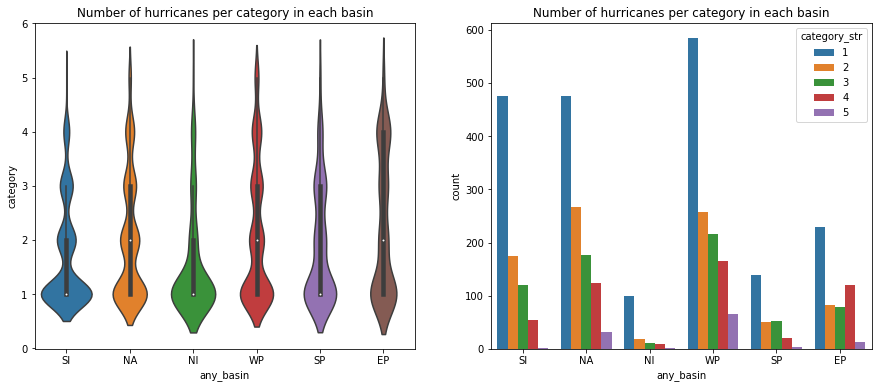We notice all basins are capable of generating major hurricanes (over 3). The Eastern Pacific basin appears to have a larger than the proportional number of major hurricanes. Below, we will regress the hurricane category against time to observe if there is a positive trend.

In :
```kde_regplot = sns.jointplot(x='min_season', y='category',
data=hurricanes_major_basins_df, kind='kde',
stat_func=stats.pearsonr).plot_joint(sns.regplot,
scatter=False)
kde_regplot.ax_joint.set_title('Scatter plot of hurricane categories over seasons')
```
Out:
`Text(0.5,1,'Scatter plot of hurricane categories over seasons')`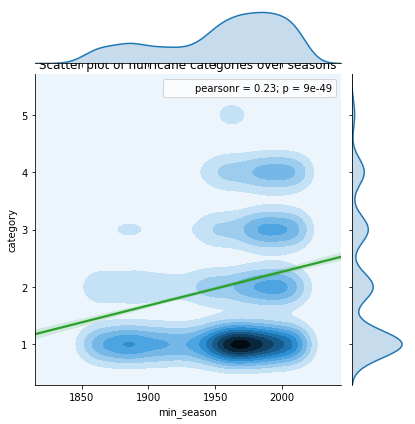Even at a global level, we notice a strong positive correlation between hurricane category and seasons. Below, we will split this across basins to observe if the trend holds well.

In :
```wgrid = sns.lmplot('min_season', 'category', col='any_basin',
data=hurricanes_major_basins_df, col_wrap=3,
sharex=False, sharey=False, line_kws={'color':'green'})
```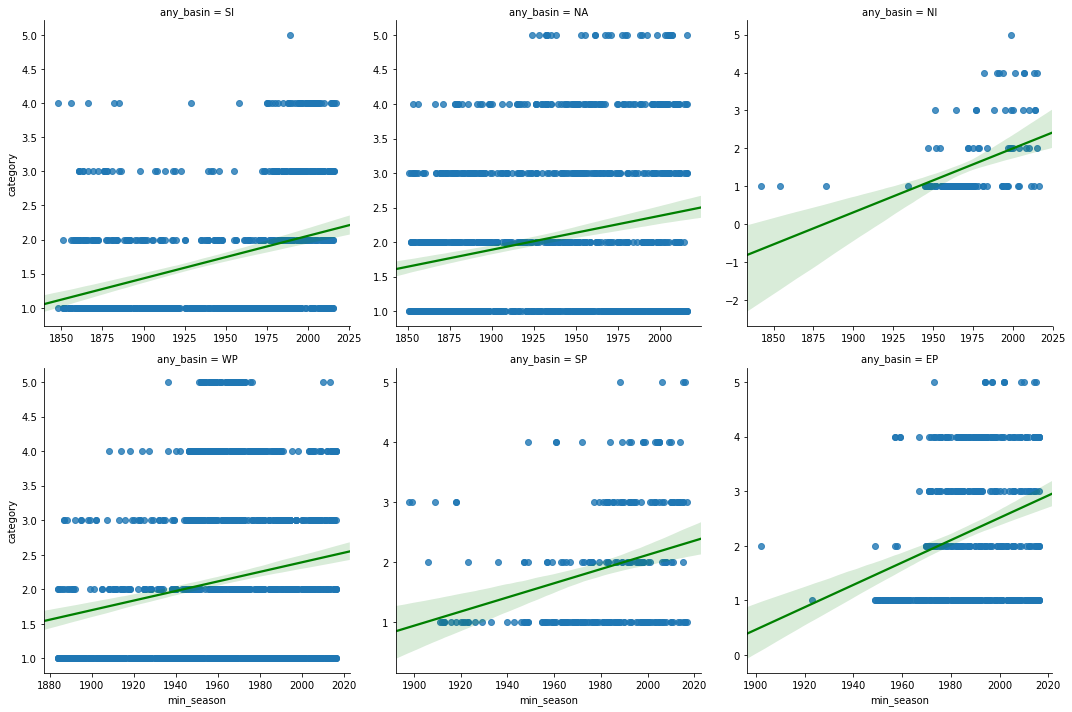In :
```category_corr_df = correlate_by_basin('min_season','category', df=hurricanes_major_basins_df)
print('Correlation coefficients for min_season vs hurricane category')
category_corr_df
```
```Correlation coefficients for min_season vs hurricane category
```
Out:
pearson-r p-value
SI 0.3314 0.0
NA 0.2161 0.0
NI 0.4612 0.0
WP 0.1849 0.0
SP 0.2908 0.0
EP 0.2919 0.0

Thus, at both global and basin scales, we notice a positive trend in the number of hurricanes of category `4` and higher, while there is a general reduction in the quantity of hurricanes per season. This is along the lines of several studies    . Thus while the total number of hurricanes per season may reduce, we notice an increase in the intensity of them.

## Does eye pressure decrease over time?¶

Just like a high wind speed, lower atmospheric pressure increases the intensity of hurricanes. To analyze this, we produce a scatter grid of `min_pressure_merged` column and regress it against `min_season` column. We split this by basins.

In :
```pgrid = sns.lmplot('min_season', 'min_pressure_merged', col='any_basin',
data=hurricanes_major_basins_df, col_wrap=3,
sharex=False, sharey=False, line_kws={'color':'green'})
```